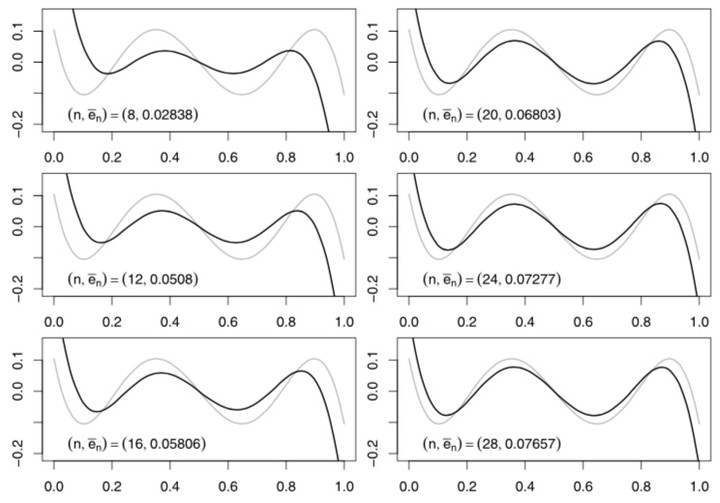# Approximation of Stochastic Programming ProblemsChebyshev minimizers over grids of points

In Stochastic Programming, Statistics or Econometrics, one often looks for the solution of optimization problems of the following form: \begin{equation} \inf_{\theta\in\Theta} \mathbb{E}_{\mathbb{P}_{}} g(\cdot,\theta)=\inf_{\theta\in\Theta} \int_{\mathbb{R}^{q}}g(y,\theta)\mathbb{P}_{}(dy) \end{equation} where $\Theta$ is a Borel subset of $\mathbb{R}^{p}$ and $\mathbb{P}$ is a probability measure defined on $\mathbf{Y}=\mathbb{R}^{q}$ endowed with its Borel $\sigma$-field $\mathcal{B}(\mathbf{Y})$ (but more general spaces can be considered). Most of the time, the mean functional $\mathbb{E}_\mathbb{P},g(\cdot,\theta)$ cannot be explicitly or easily calculated. Fortunately, there are situations where it is possible to approximate the original problem by a sequence of more tractable problems where $\mathbb{P}$ is replaced with a probability $\mathbb{P}_{n}$. In this approximation process, it is expected that the optimization problem relative to $\mathbb{P}_{n}$, namely, \begin{equation} \inf_{\theta\in\Theta}\mathbb{E}_{\mathbb{P}_{n}} g(\cdot,\theta)=\inf_{\theta\in\Theta}\int_{\mathbb{R}^{q}}g(y,\theta)\mathbb{P}_{n}(dy) \end{equation} be simpler than the original one and that the sequence $\mathbb{P}_{n}$ converge to $\mathbb{P}$ in some sense, for example in the sense of narrow convergence. If a sample of realizations of the random variable $Y$ is available, the expectation can be approximated through the empirical mean. This is the basis of the so-called $M$-estimation used in Statistics and Econometrics, that is, estimation obtained by optimizing a function of the sample with respect to one or several parameters and of the sample average approximation method used in Stochastic Programming. This project aims at studying conditions under which the solution of the original problem can be approximated by the solution of the approximating problem.##### Raffaello Seri
###### Professor of Econometrics

My research interests include statistics, numerical analysis, operations research, psychology, economics and management.